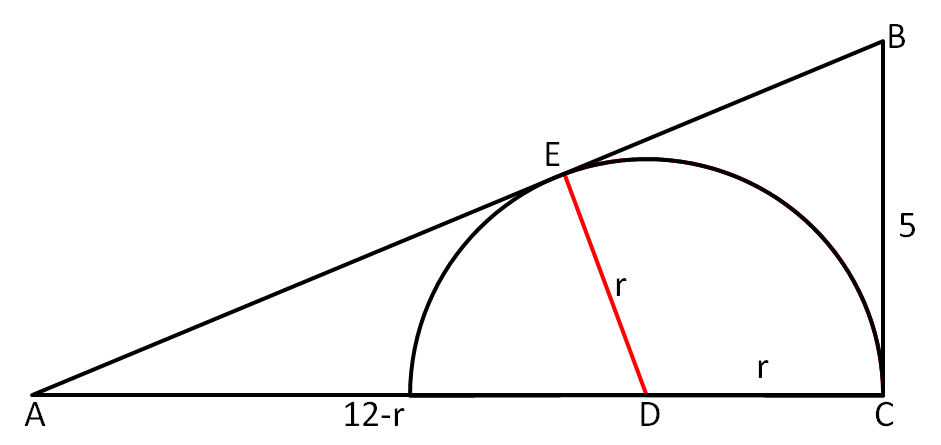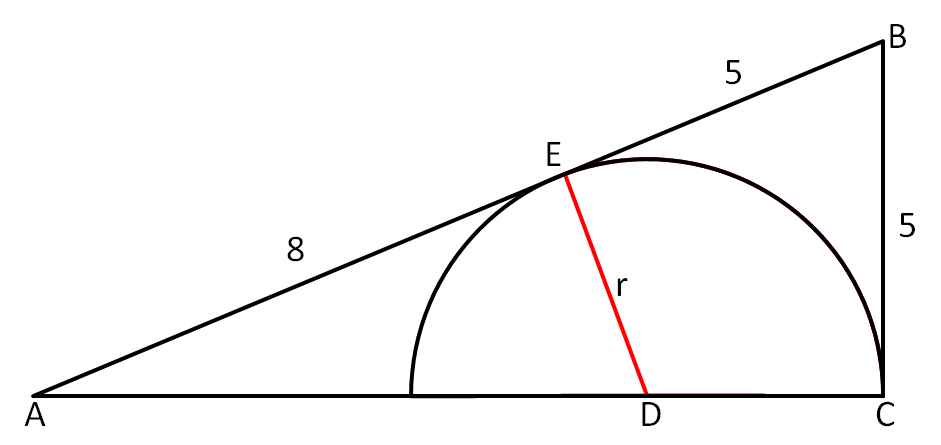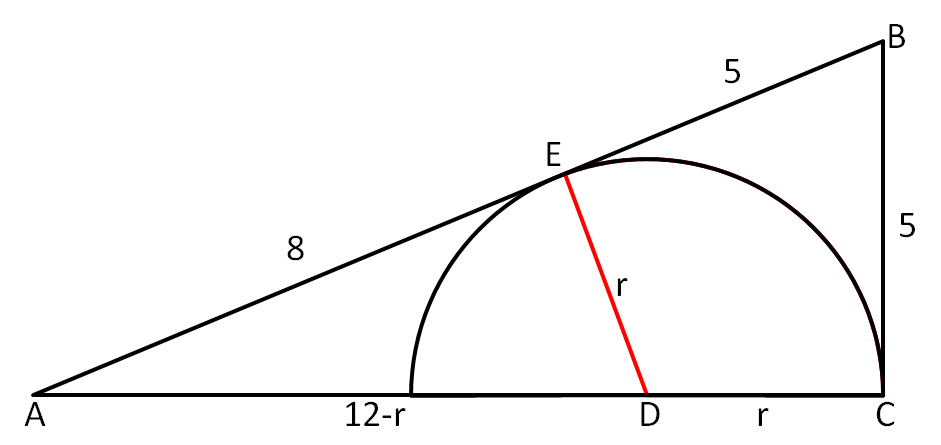#### You may also like### Some(?) of the Parts

A circle touches the lines OA, OB and AB where OA and OB are perpendicular. Show that the diameter of the circle is equal to the perimeter of the triangle### Triangle Midpoints

You are only given the three midpoints of the sides of a triangle. How can you construct the original triangle?### Fermat's Poser

Find the point whose sum of distances from the vertices (corners) of a given triangle is a minimum.

# Inscribed Semicircle

##### Age 14 to 16 Short Challenge Level:

There are a number of different possible ways of solving this problem, which are presented below.

Solution 1Since the semicircle touches the line $AB$, it is tangent there, so $\angle DEA = 90^\circ$. But then $\angle EAD = \angle CAB$, as these are the same angle. This means the triangles $ADE$ and $ABC$ are similar.

If the radius of the semicircle is $r$, then $DE = DC = r$ and $AD = 12-r$. Then, similarity says that:
$\frac{AB}{BC} = \frac{AD}{DE}$

Therefore:
$\frac{13}{5}=\frac{12-r}{r}$

Clearing the denominators gives:
$13r = 5(12-r)$

Expanding the brackets and collecting like terms gives:
$18r = 60$

Therefore, $r = 3\frac{1}{3} \text{cm}$.

Solution 2

Again, write $r$ for the radius of the semicircle.As in solution 1, we can prove that $ADE$ is similar to $ABC$. Since the semicircle touches the line $AB$, it is tangent there, so $\angle DEA = 90^\circ$. But then $\angle EAD = \angle CAB$, as these are the same angle. This means the triangles $ADE$ and $ABC$ are similar.

Then, since $\angle DCB$ is a right angle, also, $CB$ and $EB$ are both tangents of the semicircle. Since they intersect, $EB = CB = 5$, so $AE = 13-5=8$.

Then, similarity says:
$\frac{DE}{AE}=\frac{BC}{AC}$

Therefore:
$\frac{r}{8}=\frac{5}{12}$

Multiplying by $8$ gives:
$r = \frac{40}{12} = 3\frac{1}{3}$

This means the semicircle has radius $3\frac 13 \text{cm}$.

Solution 3

This approach uses Pythagoras' theorem, rather than similarity, to solve the problem. Write $r$ for the radius of the semicircle.Since $AB$ is tangent to the semicircle at $E$, $\angle AED$ is a right angle.

Also, as $BC$ is tangent to the semicircle at $C$, as $\angle ACD$ is a right angle, the lengths $BC$ and $BE$ are the same length, so both are $5\text{cm}$.

This then means that $AE = 8$, $DE = r$ and $AD = 12-r$.

Now, Pythagoras' theorem says that, since $\angle AED$ is a right angle:
$AD^2 = AE^2 + DE^2$

That is:
$(12-r)^2 = 8^2 + r^2$

Expanding the brackets gives:
$r^2 - 24r+144 = r^2 + 64$

Then, cancelling terms gives:
$24r = 80$

Therefore, $r = \frac{24}{80} = 3\frac 13$, so the radius of the semicircle is $3\frac 13\text{cm}$.

This problem is taken from the UKMT Mathematical Challenges.
You can find more short problems, arranged by curriculum topic, in our short problems collection.